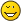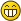MyBB Community Forums

Full Version: Best method to write a calculator on a page?
You're currently viewing a stripped down version of our content. View the full version with proper formatting.
What's the cleanest/most server/page efficient option for me to write a simple calculator?

About a dozen or so numeric fields to do some simple arithmetic on and return 3 or 4 other results.
If a JavaScript/CSS calculator is what you want to build, you're in luck. The calculator project seems to be a popular one for teaching JavaScript. Also, there are many drop-in JS calculators out there.

I've seen those and they're not quite what i want to do (and I didn't explain myself very well either, so apologies).

It's a calculation that I want to produce, not a reproduction calculator. About 10 or so numeric fields of user input, apply some fairly basic maths to get 3 or 4 different returns and display back.

It's the kind of thing you'd knock up in Excel in about 2 mins.
The simplest way, at least in my opinion, would be to use PHP to display the appropriate fields wrapped in a form and when submitted, process the values and output the results.
(03-27-2017, 09:34 PM)Wildcard Wrote: [ -> ]The simplest way, at least in my opinion, would be to use PHP to display the appropriate fields wrapped in a form and when submitted, process the values and output the results.

I was afraid you were going to say that!Oh well, gonna have to learn sometime, got any PHP for Dummies going spare?I can give you a bare-bones templates for a MyBB page:

<?php

define('IN_MYBB', 1);
define('THIS_SCRIPT', 'calculate.php');

require_once './global.php';

if (\$mybb->request_method = 'post') {
// calculate here
\$result = (int) \$mybb->input['value1'] + (int) \$mybb->input['value2];
}

\$html = <<<EOF
<html>
<body>
<div>
{\$result}
</div>
<form method="post">
<input type="text" name="value1" />
<input type="text" name="value2" />
<input type="submit" value="Calculate" />
</form>
</body>
</html>
EOF;

output_page(\$html);
exit;

?>

I didn't even test this. It is just to get you started.
(03-27-2017, 10:13 PM)Wildcard Wrote: [ -> ]I can give you a bare-bones templates for a MyBB page:

<?php

define('IN_MYBB', 1);
define('THIS_SCRIPT', 'calculate.php');

require_once './global.php';

if (\$mybb->request_method = 'post') {
// calculate here
\$result = (int) \$mybb->input['value1'] + (int) \$mybb->input['value2];
}

\$html = <<<EOF
<html>
<body>
<div>
{\$result}
</div>
<form method="post">
<input type="text" name="value1" />
<input type="text" name="value2" />
<input type="submit" value="Calculate" />
</form>
</body>
</html>
EOF;

output_page(\$html);
exit;

?>

I didn't even test this. It is just to get you started.

That's beer worthy - thank you!  I'll let you know how I get on.